## Thursday, June 13, 2013

### Lowest Common Ancestor in a Binary Tree

Another popular interview problem and the start of my tree review is finding the LCA in a binary tree. Now, pay attention - not a binary search tree, that is a different case and will be handled in a separate article; now will use a simple binary tree. For those who don't remember a binary search tree is a sorted binary tree where the left child is always smaller than the root and the right child is always larger. A simple binary tree, as in our case, doesn't have that property.

Let's use the tree below as an example.Fig.1 - Simple Binary Tree

Now let's say we want to find the LCA for nodes 4 and 9, we will need to traverse the whole tree to compare each node so that we can locate the nodes. Now considering that we start the traversal with the left branch (pre-order traversal) - we have the worst case here with O(n) running time.
Traversing the tree we compare the current node with both of the nodes and if one of them match, it means that one is the LCA on the respective branch. Let's say after traversing the above tree in pre-order  the first node that matches our nodes is 9 (2, 7, 2, 6, 5, 11, 5, 9). So the first obvious thought is that the 4 must be a child of 9, since we're already on the right child of node 5 and the pre-order traversal looks at the node first, then the left child and lastly the right child. Then we note node 9 as the LCA and we don't have to look further anymore.

Let's use another case, say we're looking for the LCA of 7 and 9. The first node in our pre-order traversal (2, 7, 2, 6, 5, 11, 5, 9, 4) is 7. Now here we can say that the LCA for the left branch is 7 because again, if the second node is in the same branch, independently of where and how deep it will be in this branch, the LCA will still be 7; thus we don't have to look in this branch anymore. But we still did not look at the right branch, so we keep traversing in a pre-order manner, but now omitting the other nodes: 2, 7, 5, 9. Now we can say that the LCA for that branch is 9. We can also affirm that the LCA for the branch with the root in node 5 is also 9. And in the end we have our nodes both in separate branches, which means that the LCA is the root of those branches - node 2.

The algorithm looks as a modified version of a pre-order tree traversal :
For the node representation we'll use the following class:

1.Tree Service Birmingham Alabama

2.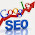Marvelous posting! I quite enjoyed reading it, you may be a great author.I will ensure that I bookmark your blog and will eventually come back in the foreseeable future..
Tree Service Company in Birmingham

3.Hi, could you please explain it a bit more. I did not understand the second example you gave.

1.4.This will not work if either of the two searched values is repeated. Say I am searching for 7, 9; and say the left subtree at root contains 7 and 9; and the right subtree (of root) contains just 9. You code returns the root as answer, where as the answer lies within the root's left subtree.

1.No, 7 and 9 should return root. I fails for 7 and 2, 5 and 9, etc

5.for the first example (finding LCA for 9 and 4), the LCA should be 5 as per my knowledge but your code give answer as 9.

6.if (root.equals(a) || root.equals(b)) {
// if at least one matched, no need to continue
// this is the LCA for this root
return root;
}

This assumption many not be valid always. For example, you maybe able to find a, but if b is not present anywhere in the tree, then u still return return a assuming it to be the ancestor anyway..

If a is present as a left child of any node, due to the last step:
return l != null ? l : r;

it will return a as the common ancestor, which it is not !!!

1.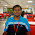We'll be given two key values no? first search and find two nodes having those key values. if we can't find either of two or both, we do not call the above function :)

2.This breaks on the case that only one match is found - which will return that one match as the common ancestor.

The problem is that the recursion uses true for both 1 match found as well as both matches found.

7.The above method fails in the example tree when searching for 7 and 2

8.I think the algorithms given will fail if you try to find LCA for two sibling nodes. for example 2 and 6 in your given example.

1.You are right Azure. It fails for the sibling nodes 2 and 6 because it returns as soon as the node is matched.

2.The algorithm works fine for the siblings too because it returns the value to the "left". Next, it repeats for the right as well. Based on the result it gives the result.

9.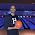This is my runnable code! :D

public class NodeTree{

public NodeTree left, right;
public int num;

public NodeTree(int n){ num = n};

}

public class question{

public static void main (String [] args) {

NodeTree n1 = new NodeTree(1);
NodeTree n2 = new NodeTree(2);
NodeTree n3 = new NodeTree(3);
NodeTree n4 = new NodeTree(4);
NodeTree n5 = new NodeTree(5);
NodeTree n6 = new NodeTree(6);
NodeTree n7 = new NodeTree(7);
NodeTree n8 = new NodeTree(8);
NodeTree n9 = new NodeTree(9);

n1.left = n2;
n1.right = n3;

n2.left = n5;
n2.right = n6;

n3.right = n4;

n5.left = n7;
n5.right = n8;

n7.left = n9;

System.out.println(commonAncestor(n1, 9, 6));

}

public static int commonAncestor(NodeTree root, int in1, int in2){

findAncestor(in1, root, stack, ancQ1);

stack.clear();
// stack = new ArrayList<>();

findAncestor(in2, root, stack, ancQ2);

int commonAns = -1;
boolean keepChecking = true;
int minSize = Math.min(ancQ1.size(), ancQ2.size());

for(int i = 0; i < minSize && keepChecking; i++){
NodeTree n1 = ancQ1.removeFirst();
NodeTree n2 = ancQ2.removeFirst();
if (n1.num == n2.num)
commonAns = n1.num;
else
keepChecking = false;
}

return commonAns;
}

public static void findAncestor(int n, NodeTree root, LinkedList stack, LinkedList ancQ){

Set visited = new HashSet<>();

while(!stack.isEmpty()){

return;

stack.removeFirst();
}
else

stack.removeFirst();
ancQ.removeLast();
}
else{

if(head.left != null ){

}

}

}
}
}

10.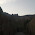My solution:

static Node lca(Node root,int v1,int v2)
{
Node currentAncestor = null;
Queue q = new LinkedList();
while(!q.isEmpty()){
Node temp = (Node)q.remove();
if( isAncestor(temp,v1) && isAncestor(temp,v2) ){
currentAncestor = temp;
}
//System.out.println(".....");
}

return currentAncestor;
}

static boolean isAncestor(Node node, int v){

if(node == null)
return false;
//System.out.println(node.data);
if(node.data == v){
return true;
}
return isAncestor(node.left, v) || isAncestor(node.right, v);
}

11.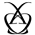This is a great analysis. I also has an in-depth discussion about this question here http://bit.ly/29kAkXM. Any comment is welcomed :)

12.13.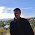thanks for explaining in easy way